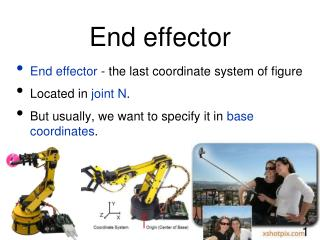DownloadDownload PresentationEnd effector

# End effector

Download Presentation## End effector

- - - - - - - - - - - - - - - - - - - - - - - - - - - E N D - - - - - - - - - - - - - - - - - - - - - - - - - - -
##### Presentation Transcript

1. End effector • End effector - the last coordinate system of figure • Located in joint N. • But usually, we want to specify it in base coordinates. 1

2. End effector A transformation from the link N to the base : 2

3. End effector • We can also express it as • three rotations (around each of the coordinate axes) • followed by a translation • How can we establish a relation with the other expression ? 3

4. End effector • The originof a coordinate frame relative to some base coordinate frame is specified by the translation : 4

5. End effector • Any 3D orientation relative to some base coordinate frame can be specified by : three rotations, one around each of the coordinate axes. We do them in this order : around x, y, z. 5

6. End effector • Orientation • The roll, pitch and yaw transformation is then expressed : 7

7. End effector • Finally, thetransformation from a coordinate frame to the base frame is expressed : 8

8. End effector We obtain directly the translation vector : 9

9. End effector We can obtain the yaw angle : Because : arctan is π-periodic. Let’s use our function arctan2 to get the right angle. 10

10. End effector Knowing the yaw angle, we can obtain the pitch angle : Because : Again, let’s use our function arctan2 : 11

11. End effector We can obtain the roll angle : Because : Again, let’s use our function arctan2 : 12

12. End effector Let’s define the state vector 13

13. End effector As previously shown, The state vector is composed of elements of this matrix. It’s also a function of joint parameters : 14